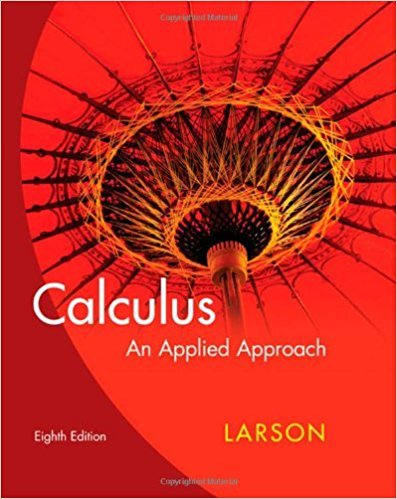×
×

# Solutions for Chapter 7.5: Extrema of Functions of Two Variables## Full solutions for Calculus: An Applied Approach | 8th Edition

ISBN: 9780618958252Solutions for Chapter 7.5: Extrema of Functions of Two Variables

Solutions for Chapter 7.5
4 5 0 277 Reviews
30
2
##### ISBN: 9780618958252

This textbook survival guide was created for the textbook: Calculus: An Applied Approach , edition: 8. Since 52 problems in chapter 7.5: Extrema of Functions of Two Variables have been answered, more than 21798 students have viewed full step-by-step solutions from this chapter. Chapter 7.5: Extrema of Functions of Two Variables includes 52 full step-by-step solutions. This expansive textbook survival guide covers the following chapters and their solutions. Calculus: An Applied Approach was written by and is associated to the ISBN: 9780618958252.

Key Calculus Terms and definitions covered in this textbook
• Absolute maximum

A value ƒ(c) is an absolute maximum value of ƒ if ƒ(c) ? ƒ(x) for all x in the domain of ƒ.

• Amplitude

See Sinusoid.

• Anchor

See Mathematical induction.

• Bias

A flaw in the design of a sampling process that systematically causes the sample to differ from the population with respect to the statistic being measured. Undercoverage bias results when the sample systematically excludes one or more segments of the population. Voluntary response bias results when a sample consists only of those who volunteer their responses. Response bias results when the sampling design intentionally or unintentionally influences the responses

• Complex plane

A coordinate plane used to represent the complex numbers. The x-axis of the complex plane is called the real axis and the y-axis is the imaginary axis

• Direction vector for a line

A vector in the direction of a line in three-dimensional space

• Exponential growth function

Growth modeled by ƒ(x) = a ? b a > 0, b > 1 .

• Implicitly defined function

A function that is a subset of a relation defined by an equation in x and y.

• Independent variable

Variable representing the domain value of a function (usually x).

• Least-squares line

See Linear regression line.

• Linear regression equation

Equation of a linear regression line

• Mean (of a set of data)

The sum of all the data divided by the total number of items

• One-to-one function

A function in which each element of the range corresponds to exactly one element in the domain

• Perihelion

The closest point to the Sun in a planet’s orbit.

• Phase shift

See Sinusoid.

An angle in standard position whose terminal side lies on an axis.

• Quotient polynomial

See Division algorithm for polynomials.

• Real part of a complex number

See Complex number.

• Real zeros

Zeros of a function that are real numbers.

• Vertex of an angle

See Angle.

×